Counting Money
Calculating Change
Bonus
200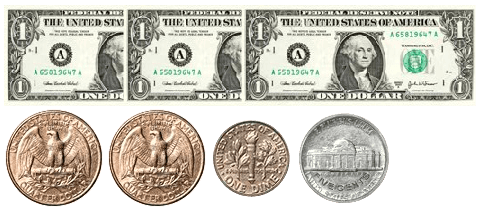$3.65 200 I used a$20 bill to buy an item that cost $5. How much change will I get back?$15

200

T/F - You should never save money.

False

400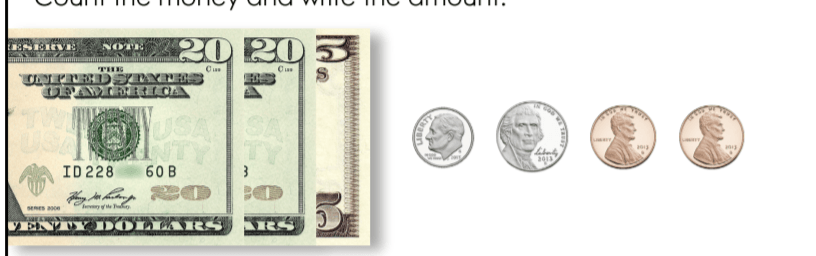How much money is shown?

$45.17 400 I used$1.00 to buy something that costs $0.50. How much change will I get back?$0.50

400

$17.89 +$26.92

What is $44.81? 600$16.66

600

How much change will you get back?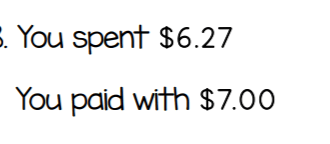What is $0.73? 600 I want to buy something that costs$64.99. How much do I need?  (use the dollar-up method)

$65 800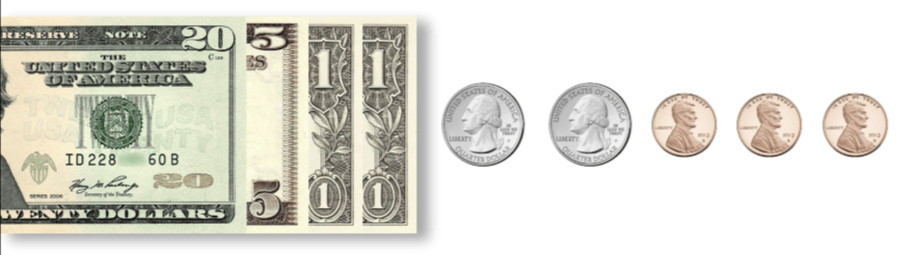$27.53

800

How much change should you get back?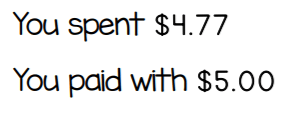What is $0.23? 800 I want to buy something that is$99.15. How much money do I need? (Use the dollar-up method)

What is \$100?

Click to zoom